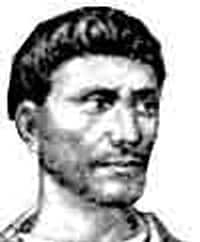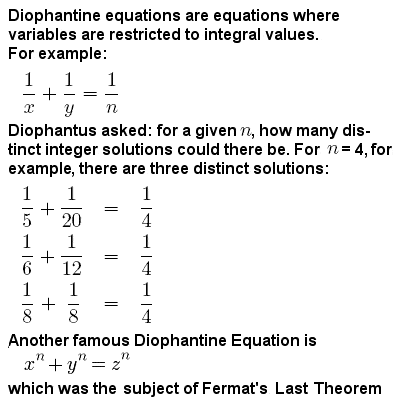# DIOPHANTUS OF ALEXANDRIA

## Biography#e8a74fDiophantus of Alexandria (c.200-284 CE)#e8a74f

Diophantus was a Hellenistic Greek (or possibly Egyptian, Jewish or even Chaldean) mathematician who lived in Alexandria during the 3rd Century CE. He is sometimes called “the father of algebra”, and wrote an influential series of books called the “Arithmetica”, a collection of algebraic problems which greatly influenced the subsequent development of number theory.

He also made important advances in mathematical notation, and was one of the first mathematicians to introduce symbolism into algebra, using an abridged notation for frequently occurring operations, and an abbreviation for the unknown and for the powers of the unknown. He was perhaps the first to recognize fractions as numbers in their own right, allowing positive rational numbers for the coefficients and solutions of his equations.

Diophantus applied himself to some quite complex algebraic problems, particularly what has since become known as Diophantine Analysis, which deals with finding integer solutions to kinds of problems that lead to equations in several unknowns.

## Diophantine equations

Diophantine equations can be defined as polynomial equations with integer coefficients to which only integer solutions are sought.#e8a74fDiophantine equations#e8a74f

For example, he would explore problems such as: two integers such that the sum of their squares is a square (x2 + y2 = z2, examples being x = 3 and y = 4 giving z = 5, or x = 5 and y =12 giving z = 13); or two integers such that the sum of their cubes is a square (x3 + y3 = z2, a trivial example being x = 1 and y = 2, giving z = 3); or three integers such that their squares are in arithmetic progression (x2 + z2 = 2y2, an example being x = 1, z = 7 and y = 5). His general approach was to determine if a problem has infinitely many, or a finite number of solutions, or none at all.

Diophantus’ major work (and the most prominent work on algebra in all Greek mathematics) was his “Arithmetica”, a collection of problems giving numerical solutions of both determinate and indeterminate equations. Of the original thirteen books of the “Arithmetica”, only six have survived, although some Diophantine problems from “Arithmetica” have also been found in later Arabic sources. His problems exercised the minds of many of the world’s best mathematicians for much of the next two millennia, with some particularly celebrated solutions provided by Brahmagupta, Pierre de Fermat, Joseph Louis Lagrange and Leonhard Euler, among others. In recognition of their depth, David Hilbert proposed the solvability of all Diophantine problems as the tenth of his celebrated problems in 1900, a definitive solution to which only emerged with the work of Robinson and Matiyasevich in the mid-20th Century.

## Epitaph

One of the problems in a later 5th Century Greek anthology of number games is sometimes considered to be Diophantus’ epitaph:

Here lies Diophantus.
God gave him his boyhood one-sixth of his life;
One twelfth more as youth while whiskers grew rife;
And then yet one-seventh ‘ere marriage begun.
In five years there came a bouncing new son;
Alas, the dear child of master and sage,
After attaining half the measure of his father’s life, chill fate took him.
After consoling his fate by the science of numbers for four years, he ended his life.

The puzzle implies that Diophantus lived to be about 84 years old (although its biographical accuracy is uncertain).